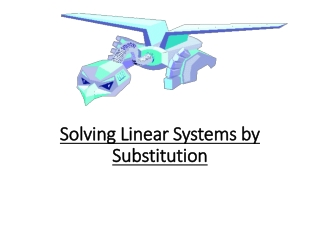DownloadDownload PresentationSolving Linear Systems by Substitution

# Solving Linear Systems by Substitution

Download Presentation## Solving Linear Systems by Substitution

- - - - - - - - - - - - - - - - - - - - - - - - - - - E N D - - - - - - - - - - - - - - - - - - - - - - - - - - -
##### Presentation Transcript

1. Solving Linear Systems by Substitution

2. Steps: • 1. Solve one of the equations for one of the variables. • Substitute that expression into the other equation and solve for the other variable. This gives you the first part of your ordered pair. • Substitute this value into the revised first equation and solve. This gives you the second part of your ordered pair. • Check the solution pair in each of the original equations. If it works, you have the solution.

3. Example: -x + y = 1 2x + y = -2 • Solve 1 equation for 1 variable. So rewrite: • -x + y = 1 • +x +x • y = x + 1 (or you could write x = y-1) • Substitute: 2x + y = -2 • 2x + (x + 1) = -2 • 2x + x + 1 = -2 • 3x + 1 = -2 • 3x = -3 • x = -1

4. Substitute: y = x + 1 • y = (-1) + 1 • y = 0 • Solution (-1, 0) • Check: -x + y = 1 • -(-1) + 0 = 1 • 1 = 1 2x + y = -2 2(-1) + 0 = -2 -2 = -2 Both are true so the solution is (-1,0). Graph to check.

5. Example: Can graph to find the approximate solution • 2x = 5 • x + y = 1 Can you tell what the solution is? Graphs do not provide precise solutions. Now solve using substitution.

6. 2x = 5 • x + y = 1 1. Solve 1 equation for 1 variable. x + y = 1 -y-y 2x = 5 2(-y + 1) = 5 -2y + 2 = 5 -2y = 3 y = -3/2 x = -y + 1 2. Now substitute–y +1into the first equation as x and solve for y. 3. Now substitute -3/2into the second equation as y and solve for x. x + y = 1 x + (-3/2) = 1 x = 5/2 or 2.5 Solution (5/2, -3/2) Check ordered pair in each equation to make sure you have the right answer!

7. Real world example: Dinner at a China Buffet Adult cost is \$11.95 Children cost \$6.95 Total bill is \$61.70 Total number of people is 6 How many adults and how many children went? Write 2 equations (one is typically a total amount of something, the other is often a price or value of the items – make sure to keep them separate to ensure you write the correct coefficients into the correct equations) A + C = 6 this equation represent the number of people, so A stands for number of adults, C is number of children, and set it = to total number of people 11.95A + 6.95C = 61.70 this equation represents cost so put the cost coefficient on each variable and have it = the total cost

8. A+C=6 rewrite as A=6-C (solve one equation for one variable) 11.95 ( 6 - C) + 6.95 C = 61.70 (substitute that into A in the other equation) 71.70 – 11.95 C + 6.95 C = 61.70 (solve for C) 71.70 – 5 C = 61.70 - 5C = -10 C = 2 A + C = 6 A + 2 = 6 A = 4 There are 4 adults and 2 children. 4(11.95) + 2(6.95) = 61.70 47.80 + 13.90 = 61.70 61.70 = 61.70 (Check)